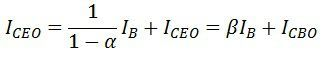A semiconductor used for amplification or for switching electronics signal is Transistor. Transistor is composed of three regions and also are of two types. Those are NPN and PNP transistors.

Let discuss about the amplification of transistor here. For amplification transistor should be properly biased. Lets see the working principle at first through the diagram.In this figure NPN transistor is used. When dc biased voltage is applied to the baseased junction then it acts as a forward biased. So by raising small input ac signal collector current increases and it acts as an amplifier. Here small change in input signal results in appreciable changes in output current.

Gain in current is given by

##### Current gain, β=ΔIC/ΔIB

This implies small input in base current results β times the input current in collector section.

The Value of β depends on transistor and normally ranges from 20 to 500.

### Relation Between Current Amplification Factor (α) & Base Amplification Factor (β)

The relation between Β and α can be derived as

Now,Substituting the value of ΔIE in equation (1), we get,The above equation shows that the when the α reaches to unity, then the β reaches to infinity. In other words, the current gain in a common emitter configuration is very high, and because of this reason, the common emitter arrangement circuit is used in all the transistor applications.

## Collector Current

In CE configuration, the input current IB and the output current IC are related by the equation shown below.If the base current is open (i.e., IB = 0). The collector current is current to the emitter, and this current is abbreviated as ICEO that means collector- emitter current with the base open.Substitute the value ΔIB in equations (1), we get,